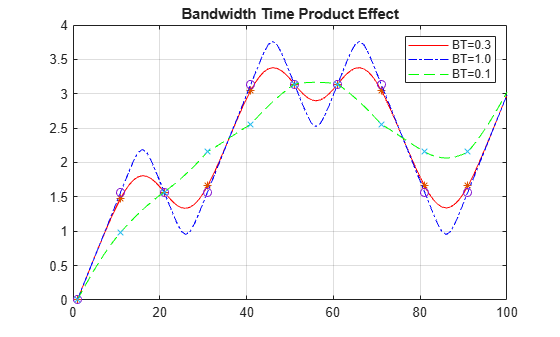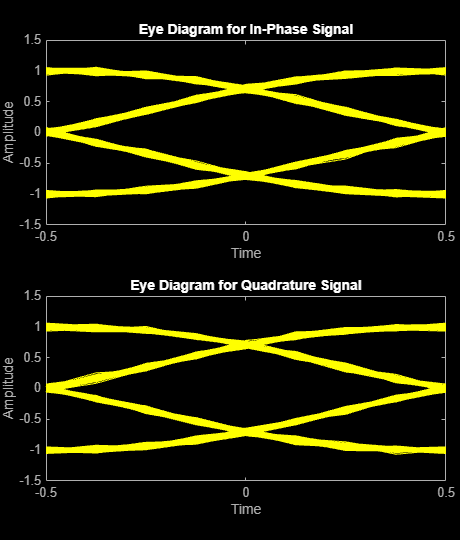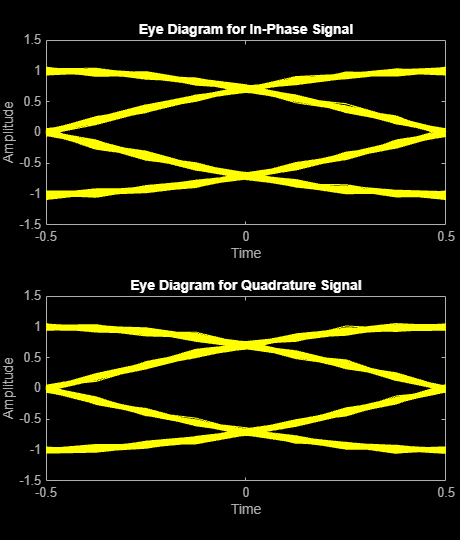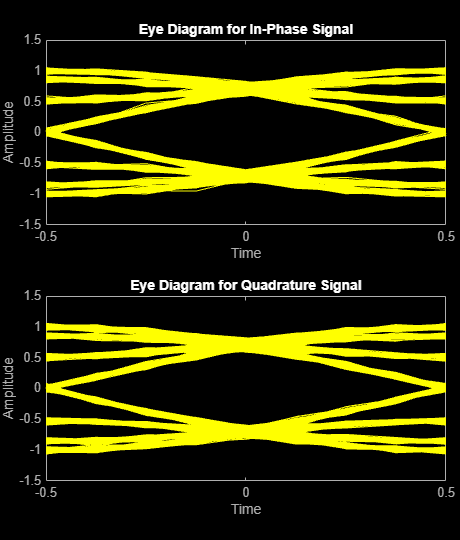# comm.GMSKModulator

Modulate using GMSK method

## Description

The `comm.GMSKModulator` System object™ modulates using the Gaussian minimum shift keying (GMSK) method. The output is a baseband representation of the modulated signal. For more detail, see Algorithms.

To modulate a signal by using the GMSK method:

1. Create the `comm.GMSKModulator` object and set its properties.

2. Call the object with arguments, as if it were a function.

## Creation

### Syntax

``gmskmodulator = comm.GMSKModulator``
``gmskmodulator = comm.GMSKModulator(Name,Value)``

### Description

example

````gmskmodulator = comm.GMSKModulator` creates a modulator System object that modulates the input signal using the GMSK modulation method.```

example

````gmskmodulator = comm.GMSKModulator(Name,Value)` sets properties using one or more name-value arguments. For example, `'PulseLength',6` specifies the length of the Gaussian pulse shape as 6 symbol intervals.```

## Properties

expand all

Unless otherwise indicated, properties are nontunable, which means you cannot change their values after calling the object. Objects lock when you call them, and the `release` function unlocks them.

If a property is tunable, you can change its value at any time.

Option to provide input in bits, specified as a numeric or logical `0` (`false`) or `1` (`true`).

• When you set this property to `false`, the input to the System object call requires a double-precision or signed integer data type column vector with values of `-1` or `1`.

• When you set this property to `true`, the input to the System object call requires a double-precision or logical data type column vector of `0`s and `1`s.

Data Types: `logical`

Product of the bandwidth and symbol time for the Gaussian pulse shape, specified as a positive scalar value. For more detail, see Algorithms.

To observe the effect of this property on the modulated signal, see the Effect of Bandwidth Time Product on GMSK Modulated Signal example.

Data Types: `double`

Pulse length, specified as a positive integer. The pulse length value represents the length of the Gaussian pulse shape in symbol intervals.

Data Types: `double`

Symbol prehistory, specified as `-1`, `1`, or a vector with elements equal those values. This property defines the data symbols that the modulator uses prior to the first call of the object, in reverse chronological order.

• A scalar value expands to a vector of length `PulseLength` – 1.

• For a vector, the length must be `PulseLength` – 1.

Data Types: `double`

Initial phase offset of the modulated waveform in radians, specified as a numeric scalar.

Data Types: `double`

Number of samples per symbol, specified as a positive integer. The number of samples per symbol represents the upsampling factor from input samples to output samples.

Data Types: `double`

Output data type, specified as either `double` or `single`.

## Usage

### Syntax

``Y = gmskmodulator(X)``

### Description

````Y = gmskmodulator(X)` applies GMSK modulation to the input data and returns the modulated GMSK baseband signal.```

### Input Arguments

expand all

Input data, specified as an integer or column vector of integers or bits.

The setting of the BitInput property determines the interpretation of the input data.

Data Types: `double` | `logical`

### Output Arguments

expand all

GMSK-modulated baseband signal, returned as a vector.

The length of the vector is equal to the number of input samples times the SamplesPerSymbol property. For more information about the output data type, see the OutputDataType property.

Data Types: `double` | `single`
Complex Number Support: Yes

## Object Functions

To use an object function, specify the System object as the first input argument. For example, to release system resources of a System object named `obj`, use this syntax:

`release(obj)`

expand all

 `step` Run System object algorithm `release` Release resources and allow changes to System object property values and input characteristics `reset` Reset internal states of System object

## Examples

collapse all

Map binary sequences of zeros and ones to the output of a GMSK modulator. This mapping also applies for MSK modulation.

Create a GMSK modulator that accepts binary inputs and has pulse length and samples per symbol values of 1.

```gmskmodulator = comm.GMSKModulator('BitInput',true,'PulseLength',1, ... 'SamplesPerSymbol',1);```

Create an input sequence of all zeros. Modulate the sequence.

```x = zeros(5,1); y = gmskmodulator(x)```
```y = 5×1 complex 1.0000 + 0.0000i -0.0000 - 1.0000i -1.0000 + 0.0000i 0.0000 + 1.0000i 1.0000 - 0.0000i ```

Determine the phase angle for each point. Use the `unwrap` function to show the trend.

`theta = unwrap(angle(y))`
```theta = 5×1 0 -1.5708 -3.1416 -4.7124 -6.2832 ```

A sequence of zeros causes the phase to shift by -π/2 between samples.

Reset the modulator. Modulate an input sequence of all ones.

```reset(gmskmodulator) x = ones(5,1); y = gmskmodulator(x)```
```y = 5×1 complex 1.0000 + 0.0000i -0.0000 + 1.0000i -1.0000 - 0.0000i 0.0000 - 1.0000i 1.0000 + 0.0000i ```

Determine the phase angle for each point. Use the `unwrap` function to show the trend.

`theta = unwrap(angle(y))`
```theta = 5×1 0 1.5708 3.1416 4.7124 6.2832 ```

A sequence of ones causes the phase to shift by +π/2 between samples.

Create a GMSK modulator and demodulator pair. Create an AWGN channel object.

```gmskmodulator = comm.GMSKModulator('BitInput',true, ... 'InitialPhaseOffset',pi/4); channel = comm.AWGNChannel('NoiseMethod', ... 'Signal to noise ratio (SNR)', ... 'SNR',0); gmskdemodulator = comm.GMSKDemodulator('BitOutput',true, ... 'InitialPhaseOffset',pi/4);```

Create an error rate calculator and account for the delay between the modulator and demodulator, caused by the Viterbi algorithm.

```errorRate = comm.ErrorRate('ReceiveDelay', ... gmskdemodulator.TracebackDepth);```

Process 100 frames of data looping through these steps.

1. Generate vectors with 300 elements of random binary data.

2. GMSK-modulate the data.

3. Pass the modulated data through the AWGN channel.

4. GMSK-demodulate the data.

5. Collect error statistics on the frames of data.

```for counter = 1:100 % Transmit 100 3-bit words data = randi([0 1],300,1); modSignal = gmskmodulator(data); noisySignal = channel(modSignal); receivedData = gmskdemodulator(noisySignal); errorStats = errorRate(data, receivedData); end```

Display the error statistics.

```fprintf('Error rate = %f\nNumber of errors = %d\n', ... errorStats(1), errorStats(2))```
```Error rate = 0.000133 Number of errors = 4 ```

This example demonstrates the effect of bandwidth time (BT) product on a GMSK modulated signal.

Create a binary data vector and apply GMSK modulation to the data.

```d = [0 1 1 0 1 0 0 1 1 1]'; a = comm.GMSKModulator(BitInput=true,SamplesPerSymbol=10)```
```a = comm.GMSKModulator with properties: BitInput: true BandwidthTimeProduct: 0.3000 PulseLength: 4 SymbolPrehistory: 1 InitialPhaseOffset: 0 SamplesPerSymbol: 10 OutputDataType: 'double' ```
```x = a(d); BTa = sprintf('BT=%2.1f',a.BandwidthTimeProduct);```

Plot the phase angles and use the `unwrap` function to show the trend better.

```plot(unwrap(angle(x)),'red-'); title('Bandwidth Time Product Effect') hold on; plot(1:10:length(x),unwrap(angle(x(1:10:end))),'*'); grid on```

Set the BT product to 1 and plot the phase angles in the same plot.

```a = comm.GMSKModulator(BitInput=true, ... SamplesPerSymbol=10,BandwidthTimeProduct=1)```
```a = comm.GMSKModulator with properties: BitInput: true BandwidthTimeProduct: 1 PulseLength: 4 SymbolPrehistory: 1 InitialPhaseOffset: 0 SamplesPerSymbol: 10 OutputDataType: 'double' ```
```x = a(d); BTb = sprintf('BT=%2.1f',a.BandwidthTimeProduct); plot(unwrap(angle(x)),'blue-.'); plot(1:10:length(x),unwrap(angle(x(1:10:end))),'o');```

Set the BT product to 0.1 and plot the phase angles in the same plot.

```a = comm.GMSKModulator(BitInput=true, ... SamplesPerSymbol=10,BandwidthTimeProduct=0.1)```
```a = comm.GMSKModulator with properties: BitInput: true BandwidthTimeProduct: 0.1000 PulseLength: 4 SymbolPrehistory: 1 InitialPhaseOffset: 0 SamplesPerSymbol: 10 OutputDataType: 'double' ```
`BTc = sprintf('BT=%2.1f',a.BandwidthTimeProduct);`

The spread of this pulse is inversely proportional to the BT product and a lower BT causes a wider spread over the bit symbol period. The peak amplitude of the pulse is directly proportional to the BT product and a lower peak amplitude causes narrower spread over the bit symbol period. As the bandwidth of the pulse decreases, the pulse duration increases.

```x = a(d); plot(unwrap(angle(x)),'green--'); plot(1:10:length(x),unwrap(angle(x(1:10:end))),'x'); legend(BTa,'',BTb,'',BTc,'') hold off;```Compare Gaussian minimum shift keying (GMSK) and minimum shift keying (MSK) modulation schemes by plotting the eye diagram for GMSK with different pulse lengths and for MSK.

Set the samples per symbol variable.

`sps = 8;`

Generate random binary data.

`data = randi([0 1],1000,1);`

Create GMSK and MSK modulators that accept binary inputs. Set the `PulseLength` property of the GMSK modulator to `1`.

```gmskMod = comm.GMSKModulator('BitInput',true,'PulseLength',1, ... 'SamplesPerSymbol',sps); mskMod = comm.MSKModulator('BitInput',true,'SamplesPerSymbol',sps);```

Modulate the data using the GMSK and MSK modulators.

```modSigGMSK = gmskMod(data); modSigMSK = mskMod(data);```

Pass the modulated signals through an AWGN channel having an SNR of `30` dB.

```rxSigGMSK = awgn(modSigGMSK,30); rxSigMSK = awgn(modSigMSK,30);```

Use the `eyediagram` function to plot the eye diagrams of the noisy signals. With the GMSK pulse length set to `1`, the eye diagrams are nearly identical.

`eyediagram(rxSigGMSK,sps,1,sps/2)``eyediagram(rxSigMSK,sps,1,sps/2)`Set the `PulseLength` property for the GMSK modulator object to `3`. Because the property is nontunable, the object must be released first.

```release(gmskMod) gmskMod.PulseLength = 3;```

Generate a modulated signal using the updated GMSK modulator object and pass it through the AWGN channel.

```modSigGMSK = gmskMod(data); rxSigGMSK = awgn(modSigGMSK,30);```

With continuous phase modulation (CPM) waveforms, such as GSMK, the waveform depends on values of the previous symbols as well as the present symbol. Plot the eye diagram of the GMSK signal to see that the increased pulse length results in an increase in the number of paths in the eye diagram.

`eyediagram(rxSigGMSK,sps,1,sps/2)`Experiment by changing the `PulseLength` parameter of the GMSK modulator object to other values. If you set the property to an even number, you should set `gmskMod.InitialPhaseOffset` to `pi/4` and update the offset argument of the `eyediagram` function from `sps/2` to `0` for a better view of the modulated signal. In order to more clearly view the Gaussian pulse shape, you must use scopes that display the phase of the signal, as described in the View CPM Phase Tree Using Simulink example.

## Algorithms

The `BandwidthTimeProduct` property represents the bandwidth multiplied by time. Use this property to reduce the bandwidth at the expense of increased intersymbol interference. The `PulseLength` property measures the length of the Gaussian pulse shape in symbol intervals. These equations define the frequency pulse shape. Bb represents the bandwidth of the pulse and T is the symbol durations. Q(t) is the complementary cumulative distribution function.

$\begin{array}{l}g\left(t\right)=\frac{1}{2T}\left\{Q\left[2\pi {B}_{b}\frac{t-\frac{T}{2}}{\sqrt{\mathrm{ln}\left(2\right)}}\right]-Q\left[2\pi {B}_{b}\frac{t+\frac{T}{2}}{\sqrt{\mathrm{ln}\left(2\right)}}\right]\right\}\\ Q\left(t\right)=\underset{t}{\overset{\infty }{\int }}\frac{1}{\sqrt{2\pi }}{e}^{-{\tau }^{2}/2}d\tau \end{array}$

For this System object, an input symbol of 1 causes a phase shift of π/2 radians, which corresponds to a modulation index of 0.5.

 Anderson, John B., Tor Aulin, and Carl-Erik Sundberg. Digital Phase Modulation. New York: Plenum Press, 1986.